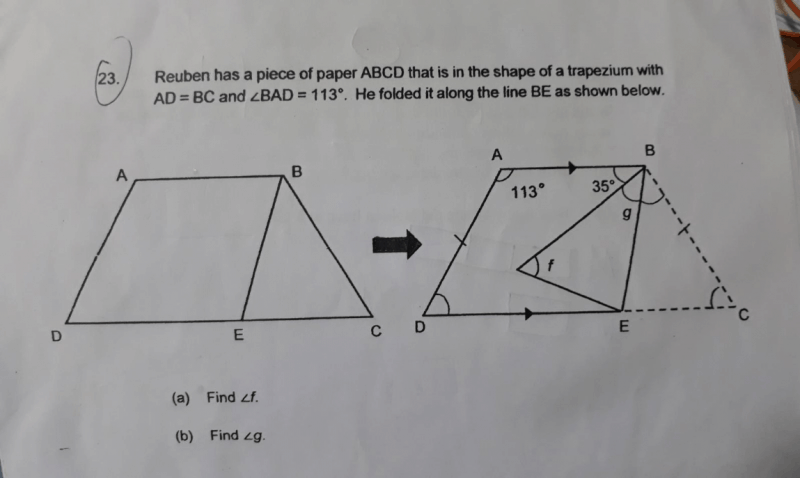# QuestionAnyone please Help with this question ?

1 Answer

# Answer

a) Since AD = BC, ∠f = ∠BCE = ∠ADE

∠f = ∠ADE
= 180° – 113°
= 67° (Interior angles between parallel lines = 180°)

b) Since AD = BC, ∠BAD = ∠ABC = 113°

∠BAC = 35° + ∠g + ∠g = 113° (∠g = ∠CBE since it is folded in)
∠g + ∠g = 113° – 35°
∠g + ∠g = 78°
∠g = 78° ÷ 2
= 39°

0 Replies 0 Likes